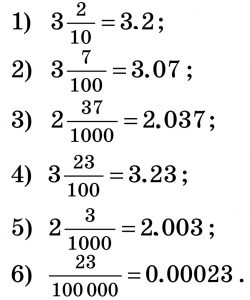# What is decimal with example

If the fraction has a denominator of 10, 100, 1000, etc., it can be written as a decimal fraction in this way: write down the whole part (if the fraction is ordinary, write 0 in place of the whole part), put a dot, and then write down the numerator of the fraction. For example:The number of digits after the dot must be equal to the number of zeros in the denominator. Therefore, to the left of the point are the digits of the integer part of the number, to the right are the digits of the fractional part, which are called decimal places.

## Properties of Decimals

If to decimal fraction add zero (or several zeros) to the right, the obtained fraction will equal to given. If the decimal fraction ends with zeros, then these zeros can be discarded. The resulting fraction is equal to the given one. For example:  0.20 = 0.2; 4.35 = 4.35000Математичні настільні ігри